# ISEE Lower Level Math : How to find the area of a parallelogram

## Example Questions

### Example Question #1 : How To Find The Area Of A Parallelogram

Find the area of the parallelogram: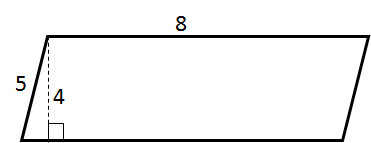Explanation: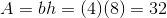### Example Question #1 : Plane Geometry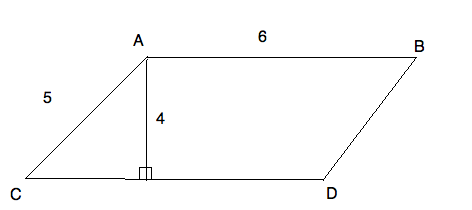What is the area of the parallelogram ABCD?Explanation:

A parallelogram's area is found by multiplying its height by the base. The height of the parallelogram is not the side. It is the line that makes a right angle with the base. Therefore, the area of this parallelogram is: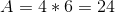### Example Question #3 : How To Find The Area Of A Parallelogram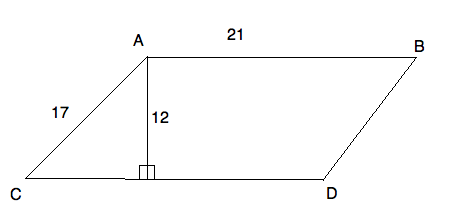What is the area of parallelogram ABCD?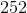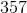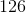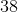Explanation:

A parallelogram's area is found by multiplying its height by the base. The height of the parallelogram is not the side. It is the line that makes a right angle with the base. Therefore, the area of this parallelogram is: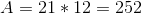### Example Question #4 : How To Find The Area Of A Parallelogram

A parallelogram measures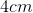along its base and is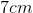high.  Calculate the area.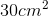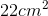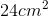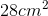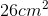Explanation:

The formula to calucate a parallelogram's area is: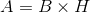You calculate the parallelogram's area by multiplying its base by its height.  Therefore,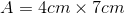### Example Question #5 : How To Find The Area Of A Parallelogram

A parallelogram measures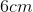along its base and is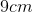high.  Calculate the area.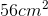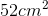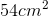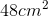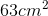Explanation:

The formula to calucate a parallelogram's area is:You calculate the parallelogram's area by multiplying its base by its height.  Therefore,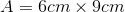### Example Question #6 : How To Find The Area Of A Parallelogram

Find the area of a parallelogram whose height isand base is.Explanation:

To solve, simply use the formula for the area of a parallelogram. Thus,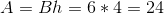### Example Question #7 : How To Find The Area Of A Parallelogram

What is the area of a parallelogram if the base is, the other side is, and the height is?Explanation:

The area of a parallelogram is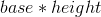so the answer would be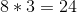.

### Example Question #31 : Geometry

Find the area of the given parallelogram: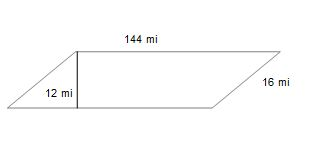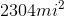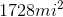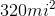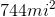Explanation:

Find the area of the given parallelogram:To find the area of a parallelogram, simply do the following: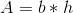Where b is the base, and h is the height. Note that the height is the length of the perpendicular line connecting both bases. In this case, our height is 12mi and our base is 144 miles.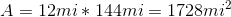### Example Question #32 : Geometry

Find the area of the given parallelogram: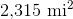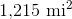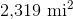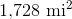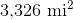Explanation:

Find the area of the given parallelogram:To find the area of a parallelogram, simply use the following formula for area of a parallelogram:Where b is the base, and h is the height. Note that the height is the length of the perpendicular line connecting both bases. In this case, our height is 12 mi and our base is 144 mi.